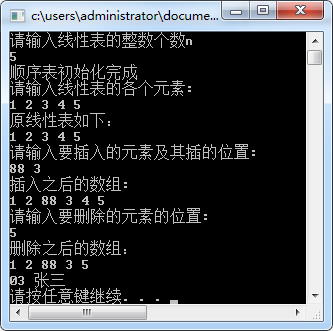2021-09-12 15:07

# 编制C/C++程序，利用顺序存储方式实现下列功能

1、 编制C/C++程序，利用顺序存储方式实现下列功能：从键盘输入数据建立一个线性表（整数），并输出该线性表；然后根据屏幕提示，进行数据的插入或删除等操作，并在插入或删除数据后输出线性表。如图所示：• 好问题 提建议
• 收藏

#### 2条回答默认 最新

•蒟蒻一枚 2021-09-12 15:58
已采纳

代码（C++）：

``````#include <bits/stdc++.h>
using namespace std;
int main() {
cout << "请输入线性表的整数个数n：\n";
int n;
cin >> n;
int *a = new int[n + 1];
cout << "顺序表初始化完成\n请输入线性表的各个元素：\n";
for (int i = 0; i < n; ++i) {
cin >> a[i];
}
cout << "原始线性表如下：\n";
for (int i = 0; i < n; ++i) {
cout << a[i] << ' ';
}
cout << "\n请输入要插入的元素及其插的位置：\n";
int k, pos;
cin >> k >> pos;
for (int i = n; i >= pos; --i) {
a[i] = a[i - 1];
}
a[pos - 1] = k;
++n;
cout << "插入之后的数组：\n";
for (int i = 0; i < n; ++i) {
cout << a[i] << ' ';
}
cout << "\n请输入要删除的元素的位置：\n";
cin >> pos;
for (int i = pos; i <= n; ++i) {
a[pos - 1] = a[pos];
}
--n;
cout << "删除之后的数组：\n";
for (int i = 0; i < n; ++i) {
cout << a[i] << ' ';
}
cout << "\n03 张三\n";
system("pause");
return 0;
}

``````

我只是个小学蒟蒻，有不清楚的地方可以私信。
回答问题不易，望采纳哦~thanks

已采纳该答案
评论
解决 2 无用
打赏 举报
•落禅 2021-09-12 15:34

我帮你解决

评论
解决 1 无用
打赏 举报